# CG NEWS & EVENTS

## VBS Meeting - After Worship!

If you are interested in volunteering for VBS this year, please stay after Worship this Sunday. We will have a short informational/planning meeting. Come see what Abundance Orchard is all about!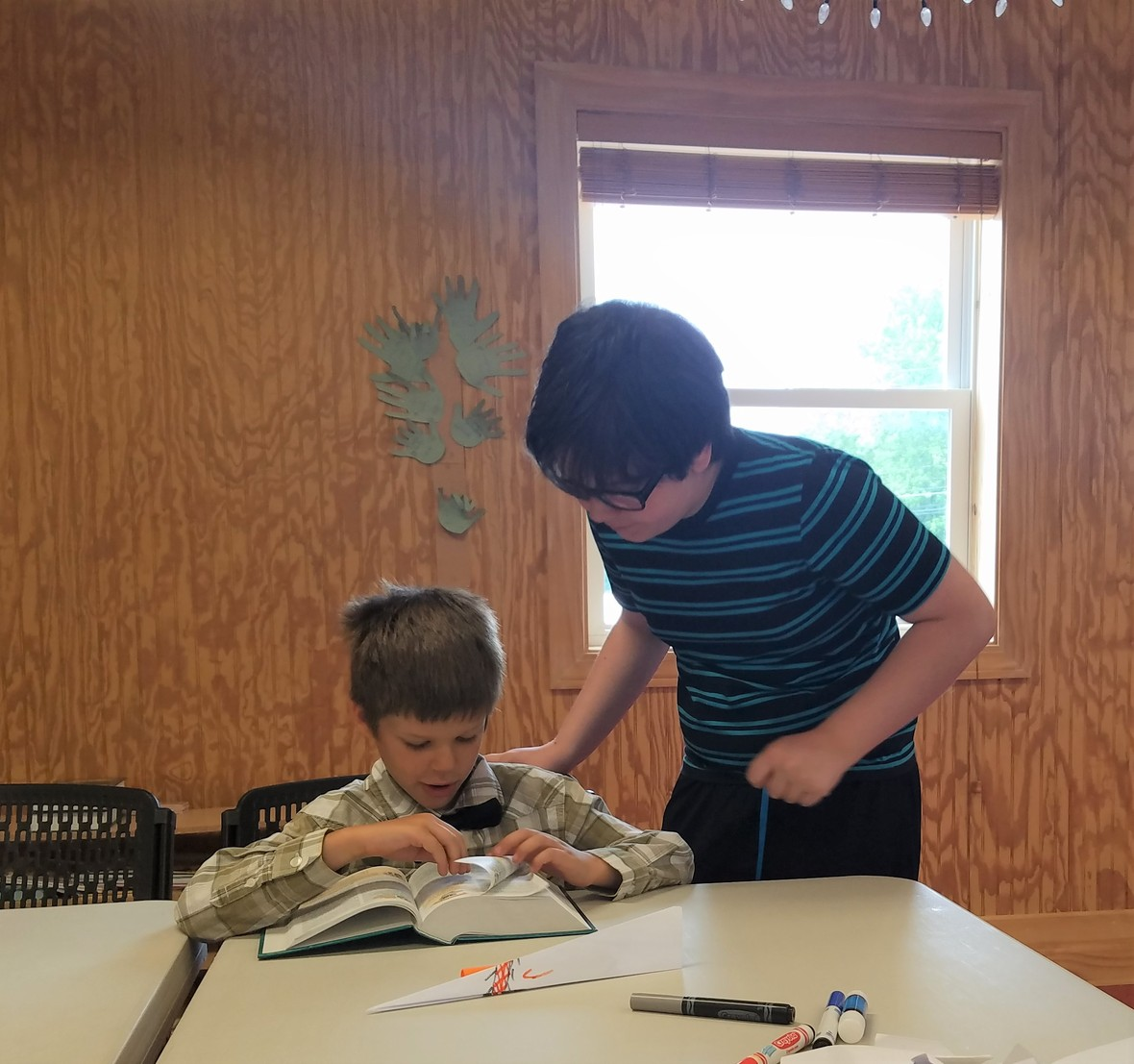## Garden News

The Garden is now on Summer Break. Our Garden age kids will remain in Worship. Activity pages will be available on the table by the Sanctuary doors. Nursery will be available for little ones through three years old.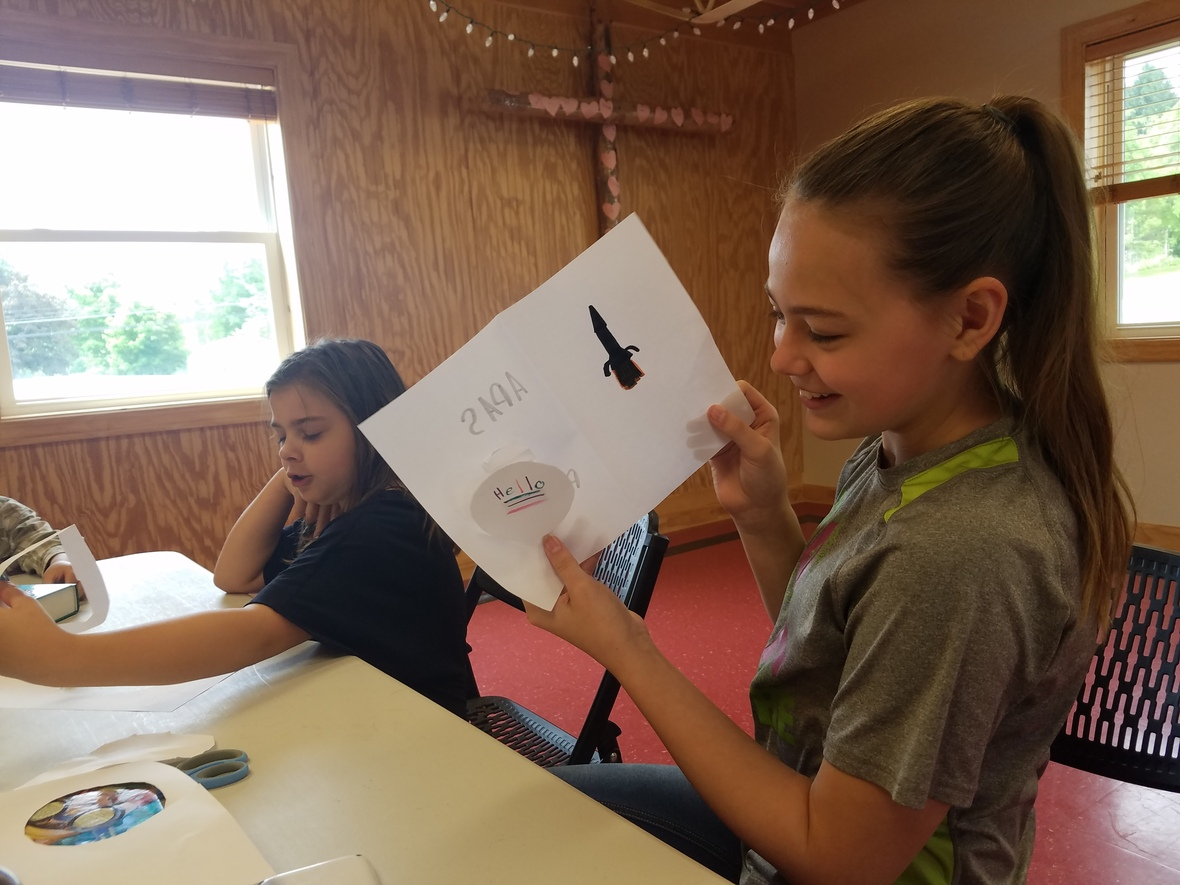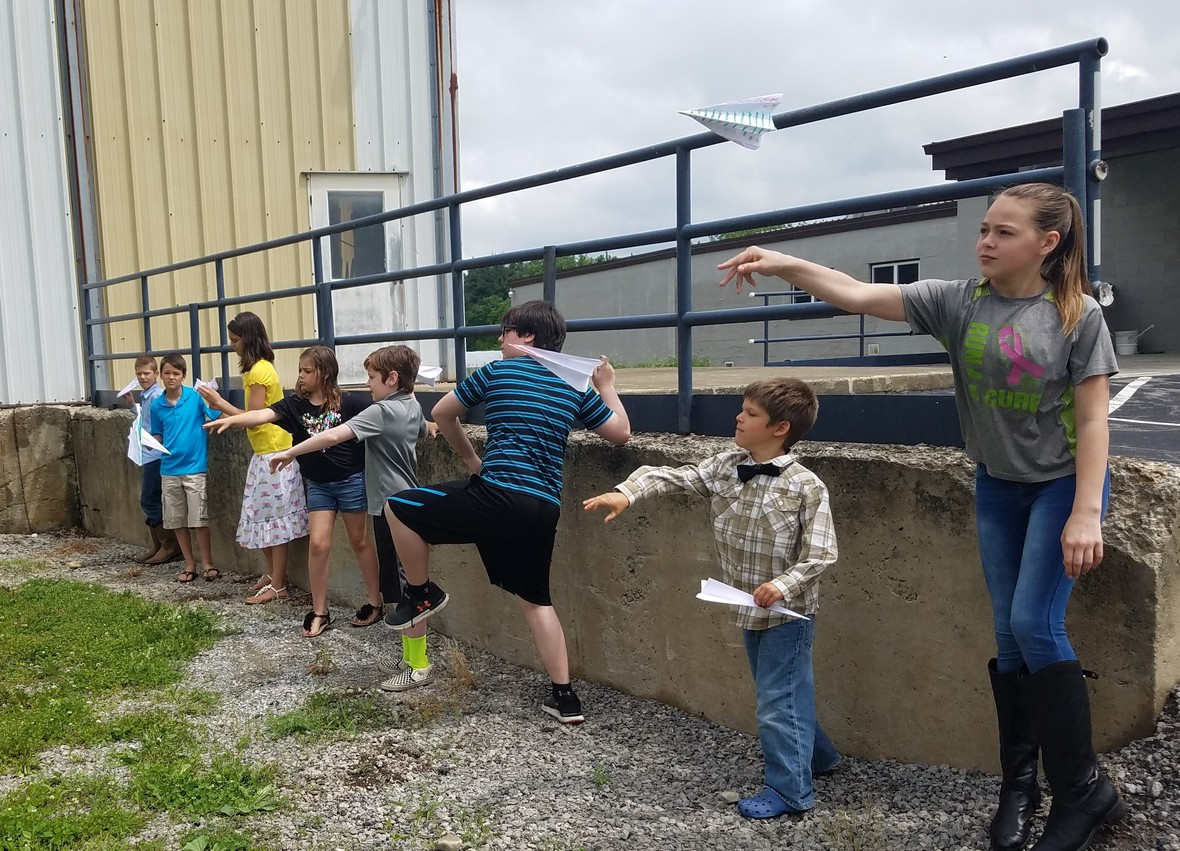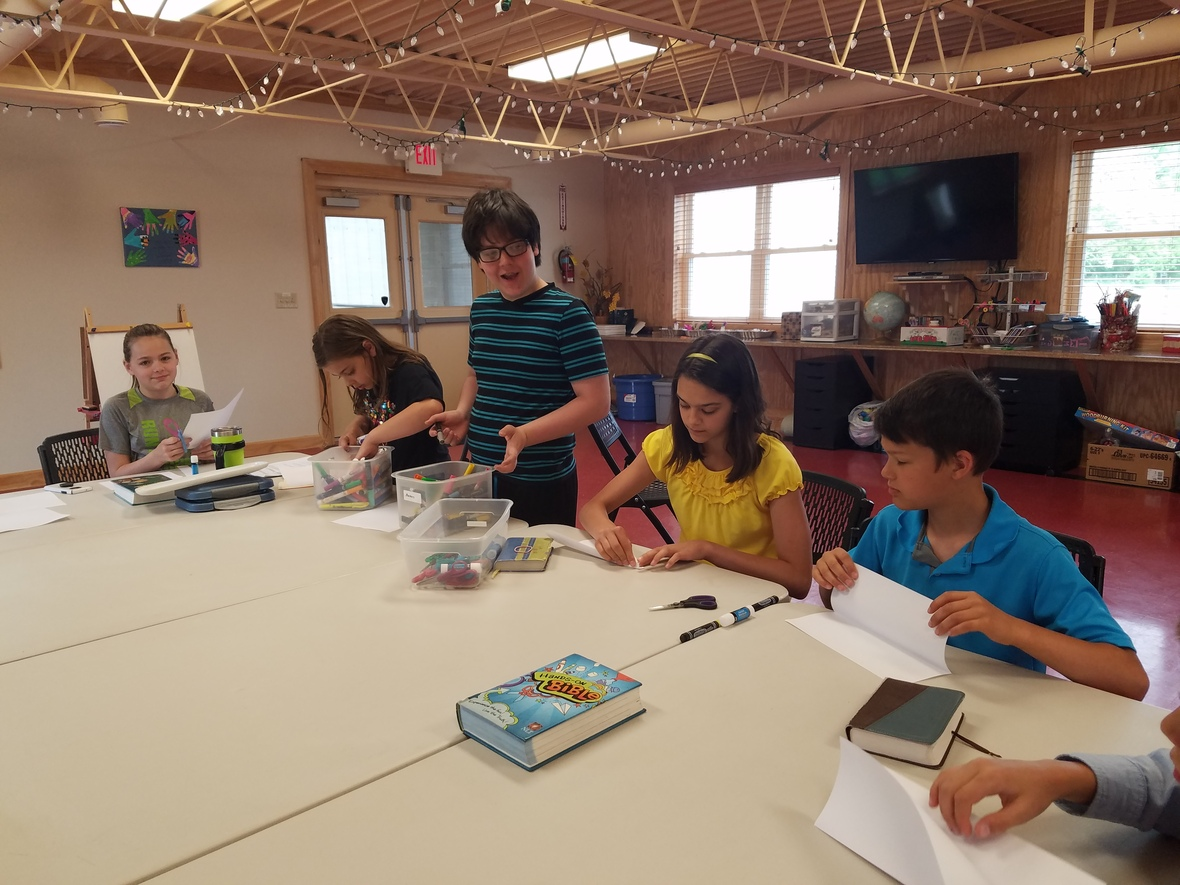## Youth Group News

Keep an eye here for our summer plans! We should have some events on the calendar by next week, including swimming at the Snyder's.

## Bible Studies

Sunday Morning: Pat Shannon leads a class on the book of Matthew; 9:30 - 10:30am.

Wednesday Morning: Ladies Study - Dwelling in God's Peace from the Jesus Calling Bible Study Series by Sarah Young; 9am.

Friday Evening: Bible Study at the Shannon's house; 6pm.

You are welcome to join in a study at any time!

# UPCOMING AT CG

## Work Day @ CG/GG - June 23

If you can help, let us know!

 table.module-12{width:0%;padding:0}table div table+table+table+table+table+table+table+table+table+table+table+table+table div table{width:0%;float:none;margin-left:auto;margin-right:auto;padding:0}table div table+table+table+table+table+table+table+table+table+table+table+table+table div table a{border:0 none;text-decoration:none}table div table+table+table+table+table+table+table+table+table+table+table+table+table div table img{width:100%!important;border:0 none;text-decoration:none}table div table+table+table+table+table+table+table+table+table+table+table+table+table div table td{width:100%;padding:0}/* styles */

## Abundance Orchard VBS

July 9 - 13 from 10:00 to 12:30

Have you put VBS week on your calendar? Invite your friends and family to join us in the orchard, where faith grows and hungry people are fed.

## GG Family Fun Fest - July 14

Help us celebrate Goodness Grows AND wrap up VBS with this annual special event! Fun for the whole family with games, face painting, special guests, and more!/* styles */

# NEWS & EVENTS

## Welcome Interns!

Our summer staff is already hard at work and we are pleased to announce the newest additions who have joined Kelly Shannon. We welcome back Trevor Wilson, a returning intern with several years experience. We also welcome Darius Surgenavic to his first summer here at GG.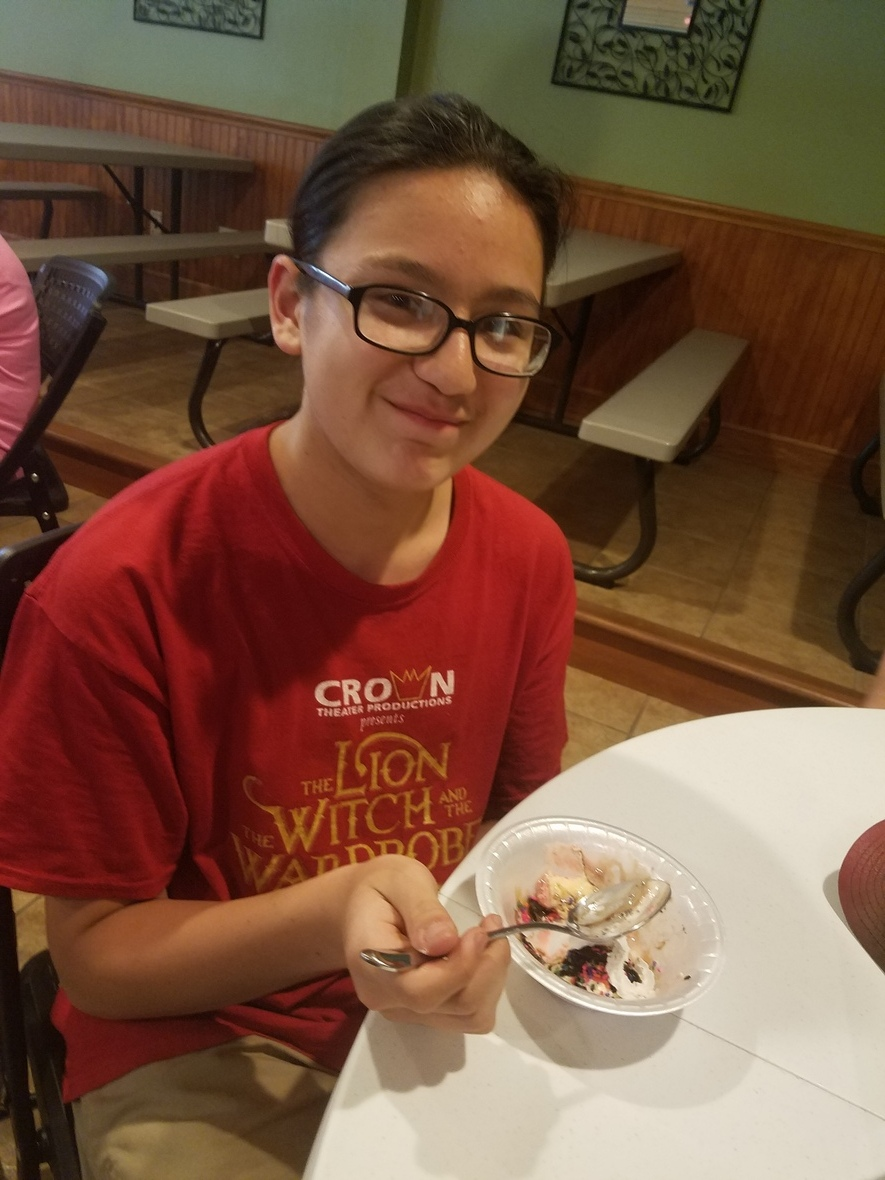## Heroes & Halos

This Saturday from 10 - 2 at Columbiana High School.

Come see us and enjoy the fun of the Heroes and Halos 7th Annual Safety Day and Interactive Health Fair! Safety services, health fair vendors, a petting zoo, food vendors, games, prizes, a giving tree, and more will be set up inside and outside. This is a fun event for the whole community, and all are welcome. We will be there making seed balls and providing samples of Jungle Jam.

## 7th Annual RiverFest

This Saturday from 11 - 4

You are invited to visit us at the B&O Station in Youngstown, for one of our favorite events of the year! We will be there with Jungle Jam and hanging baskets. Come see this beautiful location, celebrate the Mahoning River, enjoy over 20 exhibitors, listen to live music, and take a free canoe or kayak ride. This event is fun for the whole family and a great reason to take a drive to Youngstown!

## Growing Everywhere!

Tomatoes, peppers, and many more plants are now growing around the property. Our staff and clients have been digging, planting, watering, and weeding their hearts out. We send a big thank you to Mr. Don for coming in to lay the plastic and the drip irrigation in the fields! Take a walk out behind the church and explore the gardens and fields.

 table.module-23{width:0%;padding:0}table div table+table+table+table+table+table+table+table+table+table+table+table+table+table+table+table+table+table+table+table+table+table+table+table div table{width:0%;float:none;margin-left:auto;margin-right:auto;padding:0}table div table+table+table+table+table+table+table+table+table+table+table+table+table+table+table+table+table+table+table+table+table+table+table+table div table a{border:0 none;text-decoration:none}table div table+table+table+table+table+table+table+table+table+table+table+table+table+table+table+table+table+table+table+table+table+table+table+table div table img{width:100%!important;border:0 none;text-decoration:none}table div table+table+table+table+table+table+table+table+table+table+table+table+table+table+table+table+table+table+table+table+table+table+table+table div table td{width:100%;padding:0}/* styles */

## Austintown Farmers Market

Monday evenings from 5 - 8, starting June 11

The market at Austintown Township Park kicks off in just over a week! GG will be there with jams and hanging baskets. Come check out the awesome local vendors and activities for all ages that make this market the place to be every week!

## Goodness Grows News

Click right here: May Newsletter to read all about what we have been up to and what will be happening soon.

# OUTREACH and MISSION OPPORTUNITIES

## Coat & Clothing Drive

Ongoing Mission Opportunity
Drop off clothing and outerwear here, any time throughout the year. Take them to the storage room by the Cafe or up to the Green Room. Collection will continue right up until our October Giveaway Day!

 /* styles */ For I was hungry and you gave me food; I was thirsty and you gave me a drink; I was a stranger and you took me in; I was naked and you clothed me; I was sick and you visited me. I was in prison and you came to me. .....When you did it to one of the least of these my brothers and sisters, you were doing it to me. Matthew 25: 35-36, 40 ~ What can YOU do? ~~~
 table div table+table+table+table+table+table+table+table+table+table+table+table+table+table+table+table+table+table+table+table+table+table+table+table+table+table+table+table+table+table div table{width:100%;padding:0}table div table+table+table+table+table+table+table+table+table+table+table+table+table+table+table+table+table+table+table+table+table+table+table+table+table+table+table+table+table+table div table img{width:96.23%;padding:0;float:none}table div table+table+table+table+table+table+table+table+table+table+table+table+table+table+table+table+table+table+table+table+table+table+table+table+table+table+table+table+table+table div table td{width:100%;padding:0 1.88% 18px}/* styles */# LOCAL COMMUNITY NEWS/EVENTS

## Columbiana Block Party

Enjoy summer on Main Street in Columbiana. The first event is this Friday. Explore all the town has to offer. Weather permitting, Goodness Grows will be there!

## Concerts Under the Dome @ Columbiana First Presbyterian Church

 table.module-33{width:0%;padding:0}table div table+table+table+table+table+table+table+table+table+table+table+table+table+table+table+table+table+table+table+table+table+table+table+table+table+table+table+table+table+table+table+table+table+table div table{width:0%;float:none;margin-left:auto;margin-right:auto;padding:0}table div table+table+table+table+table+table+table+table+table+table+table+table+table+table+table+table+table+table+table+table+table+table+table+table+table+table+table+table+table+table+table+table+table+table div table a{border:0 none;text-decoration:none}table div table+table+table+table+table+table+table+table+table+table+table+table+table+table+table+table+table+table+table+table+table+table+table+table+table+table+table+table+table+table+table+table+table+table div table img{width:100%!important;border:0 none;text-decoration:none}table div table+table+table+table+table+table+table+table+table+table+table+table+table+table+table+table+table+table+table+table+table+table+table+table+table+table+table+table+table+table+table+table+table+table div table td{width:100%;padding:0}/* styles */

## Crown Theater Productions June Events

June 1st ~ Free Movie Double Feature:
Paddington 2 (PG) @ 7pm & The Greatest Showman (PG) @ 9:15pm

 /* styles */ June 4th ~ Summer Drama Camp begins
 /* styles */ June 8, 9, 10, 15, 16, 17 ~ Signin' In the Rain Fridays & Saturdays 8:00pm, Sundays 2:00pm
 /* styles */ June 23rd & 24th ~ Legally Blonde Jr. presented by the Summer Drama Camp For more information about Crown Theater, or to purchase tickets, click Here.
 /* styles */ ~~~ If you know of any interesting news or events, please let us know, so that we can pass it on to others!
 table div table+table+table+table+table+table+table+table+table+table+table+table+table+table+table+table+table+table+table+table+table+table+table+table+table+table+table+table+table+table+table+table+table+table+table+table+table+table+table+table div table{width:100%;padding:0}table div table+table+table+table+table+table+table+table+table+table+table+table+table+table+table+table+table+table+table+table+table+table+table+table+table+table+table+table+table+table+table+table+table+table+table+table+table+table+table+table div table img{width:96.23%;padding:0;float:none}table div table+table+table+table+table+table+table+table+table+table+table+table+table+table+table+table+table+table+table+table+table+table+table+table+table+table+table+table+table+table+table+table+table+table+table+table+table+table+table+table div table td{width:100%;padding:0 1.88% 18px}/* styles */# CELEBRATE!

Fresh Fruit & Vegetable Month - June!
(Fits right in with our mission of Goodness Grows!)
National Donut Day - June 2
National Hug Your Cat Day - June 4
Colin Snyder - June 6 (13!)

 table.module-41{width:0%;padding:0}table div table+table+table+table+table+table+table+table+table+table+table+table+table+table+table+table+table+table+table+table+table+table+table+table+table+table+table+table+table+table+table+table+table+table+table+table+table+table+table+table+table+table div table{width:0%;float:none;margin-left:auto;margin-right:auto;padding:0}table div table+table+table+table+table+table+table+table+table+table+table+table+table+table+table+table+table+table+table+table+table+table+table+table+table+table+table+table+table+table+table+table+table+table+table+table+table+table+table+table+table+table div table a{border:0 none;text-decoration:none}table div table+table+table+table+table+table+table+table+table+table+table+table+table+table+table+table+table+table+table+table+table+table+table+table+table+table+table+table+table+table+table+table+table+table+table+table+table+table+table+table+table+table div table img{width:100%!important;border:0 none;text-decoration:none}table div table+table+table+table+table+table+table+table+table+table+table+table+table+table+table+table+table+table+table+table+table+table+table+table+table+table+table+table+table+table+table+table+table+table+table+table+table+table+table+table+table+table div table td{width:100%;padding:0}/* styles */

# ON THE CG CALENDAR

May 31 - June 6. . .
Saturday - GG @ RiverFest & Heroes and Halostable div table+table+table+table+table+table+table+table+table+table+table+table+table+table+table+table+table+table+table+table+table+table+table+table+table+table+table+table+table+table+table+table+table+table+table+table+table+table+table+table+table+table+table+table+table+table div table{width:100%;padding:0}table div table+table+table+table+table+table+table+table+table+table+table+table+table+table+table+table+table+table+table+table+table+table+table+table+table+table+table+table+table+table+table+table+table+table+table+table+table+table+table+table+table+table+table+table+table+table div table img{width:96.23%;padding:0;float:none}table div table+table+table+table+table+table+table+table+table+table+table+table+table+table+table+table+table+table+table+table+table+table+table+table+table+table+table+table+table+table+table+table+table+table+table+table+table+table+table+table+table+table+table+table+table+table div table td{width:100%;padding:0 1.88% 18px}/* styles */